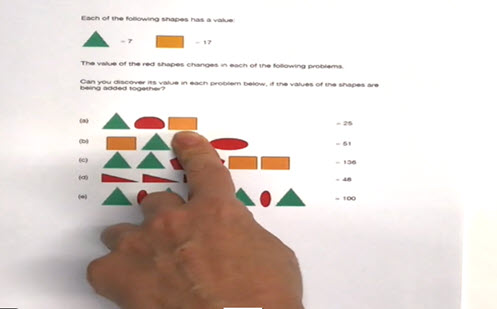Go to website

# Super shapes

Models strategies for approaching problems where multiplicative thinking and algebraic reasoning are required. Invites students to discover values of other shapes in each of the problems.

Year level(s) Year 6
Audience Student, Teacher
Purpose Teaching resource, Teaching strategies
Format Video
Teaching strategies and pedagogical approaches Concrete Representational Abstract model, Targeted teaching
Keywords addition, multiplication, reasoning, problem-solving, explicit teaching

## Curriculum alignment

Curriculum connections Numeracy
Strand and focus Number, Algebra, Build understanding
Topics Addition and subtraction, Multiplication and division, Operating with number
AC: Mathematics (V9.0) content descriptions
AC9M6N09
Use mathematical modelling to solve practical problems, involving rational numbers and percentages, including in financial contexts; formulate the problems, choosing operations and efficient calculation strategies, and using digital tools where appropriate; interpret and communicate solutions in terms of the situation, justifying the choices made

Multiplicative strategies (P8)
Understanding money (P8)